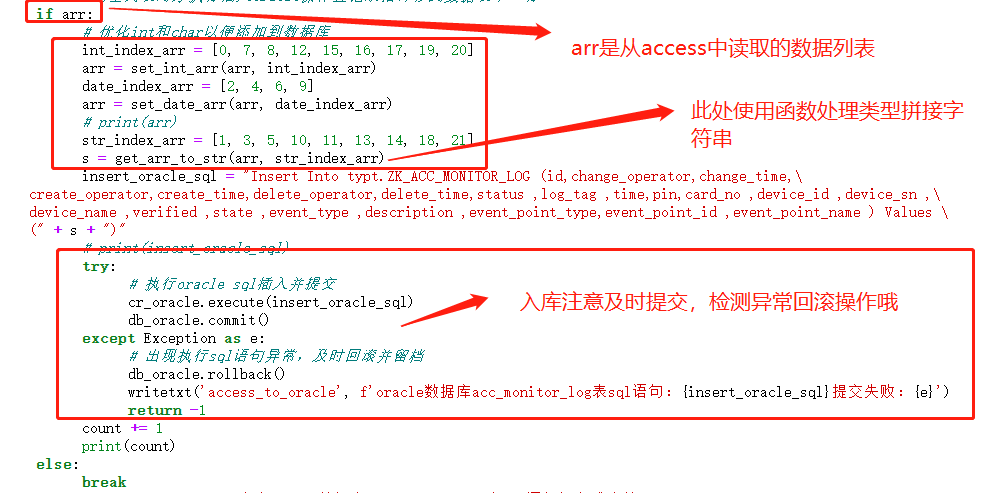# 1、熟悉 cx_Oracle

``````
import cx_Oracle
conn = cx_Oracle.connect('用户名/密码@主机ip地址/orcl')   #用自己的实际数据库用户名、密码、主机ip地址 替换即可
curs=conn.cursor()
sql='SELECT * FROM 表名' #sql语句
rr=curs.execute (sql)
row=curs.fetchone()
print(row)
curs.close()
conn.close()

``````

# 插入不同数据类型处理

## 小白函数分享

``````

def set_int_arr(arr, int_index_arr):
"""
对列表中部分数据进行整型转换
:param arr: 需要对列表中部分数据进行整型转换的列表
:param int_index_arr: 对应整型转换的列表索引位置
:return: 处理后的列表
"""
for x in range(len(arr)):
if x in int_index_arr:
if str(arr[x]) == 'None':
arr[x] = 0
else:
arr[x] = int(arr[x])
else:
pass
return arr

def set_date_arr(arr, date_index_arr):
"""
对列表中部分数据进行整型转换
:param arr: 需要对列表中部分数据进行日期时间类型转换的列表
:param date_index_arr: 对应日期时间类型转换的列表索引位置
:return: 处理后的列表
"""
for x in range(len(arr)):
if x in date_index_arr:
if arr[x] == 'None':
# 如果日期为空给予计算机初始时间作为默认值
arr[x] = "to_date('1970-01-01 00:00:00', 'yyyy-mm-dd hh24:mi:ss')"
else:
# 优化部分日期格式后含“.”或“+”
# arr[x] = arr[x].split('.')
arr[x] = f"to_date('{arr[x].split('+')}', 'yyyy-mm-dd hh24:mi:ss')"
else:
pass
return arr

def get_arr_to_str(arr, str_index_arr):
"""
对列表数据进行字符串拼接
:param arr: 要拼接到sql语句中单行数据所有字段组成的列表
:param str_index_arr: 对应字符串类型转换的列表索引位置
:return: 按指定格式拼接后的字符串
"""
s = ''
for i in range(len(arr)):
if i < len(arr) - 1:
if i in str_index_arr:
s += f"'{arr[i]}',"
else:
s += f"{arr[i]},"
else:
if i in str_index_arr:
s += f"'{arr[i]}'"
else:
s += f"{arr[i]}"
return s

``````